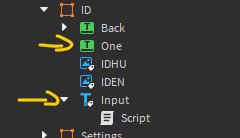# Keyboard / Textbox GUI

Hello,

I have a keyboard GUI, and how can i make the following:

If i click a number, then in the Textbox, the number i typed appears.

Thank you to anyone who can help me!

You can make this possible using a table.

``````local numbers = {
Zero = 0,
One = 1,
Two = 2,
Three = 3,
Four = 4,
Five = 5,
Six = 6,
Seven = 7,
Eight = 8,
Nine = 9
}

game:GetService("UserInputService").InputBegan:Connect(function (input, gameProcessedEvent)
if gameProcessedEvent then return end

local number = numbers[input.KeyCode.Name]

if not number then
return -- Exit function, the input was not a number.
end

-- Do something with the number
end)
``````
1 Like

It looks like this, but it doesnt work for me.

Or i just dont know where to put the scripts cause im not to good in that xd

Are there any errors in console? If so, please post a screenshot. (See Output tab.)No errors. The script you sent is in the Input.

That is the problem. `UserInputService` only works in LocalScripts. You need to put the code in a LocalScript.Ohhh, im not really good in knowing that.

I changed it into a Localscript, but when i click the Number it still doesnt appear in the TextBox

Oh! You meant clicking the number, not typing it. The way it was worded, I thought you were looking for a script that allows you to type numbers in. My mistake for misunderstanding. In that case, the script should go as follows:

``````local numbers = {
Zero = 0,
One = 1,
Two = 2,
Three = 3,
Four = 4,
Five = 5,
Six = 6,
Seven = 7,
Eight = 8,
Nine = 9
}

local UI = script.Parent.Parent
local Input = script.Parent

for _, element in ipairs(UI:GetChildren()) do
if element:IsA("TextButton") then
element.Activated:Connect(function ()
Input.Text = Input.Text .. numbers[element.Name]
end)
end
end
``````

No problem, and thank you so much!!!

I have another question, i have a button to clear the text.
But it doesnt work, cause when i click the numbers, the text Value is “” so nothing.

Can you help me with a button so if i click it, it removes all the numbers i typed in.
Thank you.